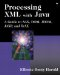# A Simple XML-RPC Client

An XML-RPC request is simply an XML document sent over a network socket. It's easy to use the techniques of this chapter to write a very simple XML-RPC client for a particular service. You just ask the user for the data to send to the server, wrap it up in some XML, and write it onto a URL object pointing at the server. The response will come back in XML as well. Since we haven't yet learned how to read XML documents, I'll just dump the response as text to System.out . Later we'll pay more attention to the response and add a nicer user interface.

The specific XML-RPC service we're going to talk to is a Fibonacci generator I'll develop in later chapters. The request passes an int to the server. The server responds with the value of that Fibonacci number. For example, the following request document asks for the value of the 23rd Fibonacci number:

` <?xml version="1.0"?>  <methodCall>   <methodName>calculateFibonacci</methodName>   <params>     <param>       <value><int>23</int></value>     </param>   </params> </methodCall> `

And here's the response:

` <?xml version="1.0"?>  <methodResponse>   <params>     <param>       <value><double>28657</double></value>     </param>   </params> </methodResponse> `

The response is passed back as a double because XML-RPC int s are limited to a maximum size of 2,147,483,647, and as previously noted Fibonacci numbers very quickly exceed that. XML-RPC does not define a maximum size for a double . Some implementations may have maximum sizes, nonetheless. If this is a concern, you can send the response back as a string instead.

The client needs to read an integer input by the user, wrap that integer in the XML-RPC envelope, then send that document to the server using HTTP POST. In Java the simplest way to post a document is through the java.net.HttpURLConnection class. You get an instance of this class by calling a URL object's openConnection() method, and then casting the resulting URLConnection object to HttpURLConnection . Once you have an HttpURLConnection , you set its protected doOutput field to true, using the setDoOutput() method; and its request method to POST, using the setRequestMethod() method. Then you grab hold of an output stream using getOutputStream() and proceed to write your request body on that. Example 3.10 does all of this.

##### Example 3.10 Connecting an XML-RPC Server with URLConnection
` import java.net.*; import java.io.*; public class FibonacciXMLRPCClient {   private static String defaultServer    = "http://www.elharo.com/fibonacci/XML-RPC";   public static void main(String[] args) {     if (args.length == 0) {       System.out.println(        "Usage: java FibonacciXMLRPCClient index serverURL");       return;     }     String index = args;     String server;     if (args.length <= 1) server = defaultServer;     else server = args;     try {       URL u = new URL(server);       URLConnection uc = u.openConnection();       HttpURLConnection connection = (HttpURLConnection) uc;       connection.setDoOutput(true);       connection.setDoInput(true);       connection.setRequestMethod("POST");       OutputStream out = connection.getOutputStream();       OutputStreamWriter wout = new OutputStreamWriter(out, "UTF-8");       wout.write("<?xml version=\"1.0\"?>\r\n");       wout.write("<methodCall>\r\n");       wout.write(        "  <methodName>calculateFibonacci</methodName>\r\n");       wout.write("  <params>\r\n");       wout.write("    <param>\r\n");       wout.write("      <value><int>");       wout.write(index);       wout.write("</int></value>\r\n");       wout.write("    </param>\r\n");       wout.write("  </params>\r\n");       wout.write("</methodCall>\r\n");       wout.flush();       out.close();       InputStream in = connection.getInputStream();       int c;       while ((c = in.read()) != -1) System.out.write(c);       System.out.println();       in.close();       out.close();       connection.disconnect();     }     catch (IOException e) {       System.err.println(e);       e.printStackTrace();     }   }  // end main } `

You'll see the servlet that services this client in Chapter 5. In the meantime, if you'd like to test this program, you can point it at http://www.elharo.com/fibonacci/XML-RPC , where I have the servlet running. Following is some sample output from that site:

` C:\XMLJAVA>  java FibonacciXMLRPCClient 10  <?xml version="1.0"?>  <methodResponse>    <params>      <param>        <value><double>55</double></value>      </param>    </params>  </methodResponse> `Processing XML with Javaв„ў: A Guide to SAX, DOM, JDOM, JAXP, and TrAX
ISBN: 0201771861
EAN: 2147483647
Year: 2001
Pages: 191